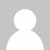So I’m still just about managing to keep up with the Advent of Code challenges. Here’s me talking through my solution to day 6’s problem:

Here’s my solution to part a done in three stages in C#

``````var instructions = File.ReadAllLines("day6.txt");

var pattern = @"(turn on|toggle|turn off)\ (\d+)\,(\d+)\ through\ (\d+)\,(\d+)";

var instructionsFlattened = instructions
.Select(i => Regex.Match(i, pattern).Groups)
.Select(g => new
{
Action = g.Value,
From = new {
X = int.Parse(g.Value),
Y = int.Parse(g.Value) },
To = new {
X = int.Parse(g.Value),
Y = int.Parse(g.Value) }
})
.SelectMany(i =>
from x in Enumerable.Range(i.From.X, 1 + i.To.X - i.From.X)
from y in Enumerable.Range(i.From.Y, 1 + i.To.Y - i.From.Y)
select new { i.Action, x, y });

// apply the instructions
var lights = new bool[1000, 1000];
foreach (var i in instructionsFlattened)
{
if (i.Action == "turn on")
lights[i.x, i.y] = true;
else if (i.Action == "turn off")
lights[i.x, i.y] = false;
else if (i.Action == "toggle")
lights[i.x, i.y] = !lights[i.x, i.y];
}

// count the lights on
(from x in Enumerable.Range(0, 1000)
from y in Enumerable.Range(0, 1000)
where lights[x, y]
select 1).Sum().Dump("lights on");
``````

But I decided I wanted to solve this in a single LINQ expression, and this is possible using LINQ’s Aggregate method, so here’s part b solved in C# with Aggregate

``````File.ReadAllLines("day6.txt")
.Select(i => Regex.Match(i,
@"(turn on|toggle|turn off)\ (\d+)\,(\d+)\ through\ (\d+)\,(\d+)").Groups)
.Select(g => new
{
Action = g.Value,
From = new { X = int.Parse(g.Value), Y = int.Parse(g.Value) },
To = new { X = int.Parse(g.Value), Y = int.Parse(g.Value) }
})
.SelectMany(i =>
from x in Enumerable.Range(i.From.X, 1 + i.To.X - i.From.X)
from y in Enumerable.Range(i.From.Y, 1 + i.To.Y - i.From.Y)
select new { i.Action, x, y })
.Aggregate(new int[1000,1000], (acc, next) => {
var brightness = acc[next.x,next.y];
if (next.Action == "turn on")
brightness+=1;
else if (next.Action == "turn off")
brightness = Math.Max(0, brightness - 1);
else if (next.Action == "toggle")
brightness += 2;
acc[next.x, next.y] = brightness;
return acc;
})
.Cast<int>() // flattens a multi-dimensional array
.Sum()
// brightness is 14687245
``````

And finally of course, as part of my on-going bid to improve my F# skills, I made an F# version of this solution:

``````let instructions = File.ReadAllLines("day6.txt")
let turnOn n = n + 1
let turnOff n = max (n - 1) 0
let toggle n = n + 2

let selectAction = function
| "turn on" -> turnOn
| "turn off" -> turnOff
| "toggle" -> toggle

let parseInstruction actionSelector i =
let pattern = @"(turn on|toggle|turn off)\ (\d+)\,(\d+)\ through\ (\d+)\,(\d+)"
let groups = Regex.Match(i, pattern).Groups
let action = actionSelector groups..Value
let fromPos = (int groups..Value), (int groups..Value)
let toPos = (int groups..Value), (int groups..Value)
(action, fromPos, toPos)

let expandPositions (x0,y0) (x1,y1) =
seq { for x in x0 .. x1 do
for y in y0 .. y1 do
yield (x,y) }

let applyAction (acc:int[,]) (action,(x,y)) =
acc.[x,y] <- action acc.[x,y]
acc

let calculate actionSelector (input:string[]) =
let startState = Array2D.create 1000 1000 0
input
|> Seq.map (parseInstruction actionSelector)
|> Seq.collect (fun (action,fromPos,toPos) ->
seq { for pos in (expandPositions fromPos toPos) do
yield (action,pos) })
|> Seq.fold applyAction startState
|> Seq.cast<int> // same trick works to flatten 2d array
|> Seq.sum

calculate selectAction instructions |> Dump

let turnOnA n = 1
let turnOffA n = 0
let toggleA n = if n = 0 then 1 else 0

let selectActionA = function
| "turn on" -> turnOnA
| "turn off" -> turnOffA
| "toggle" -> toggleA

calculate selectActionA instructions |> Dump
``````

As always, comments on ways I could improve my solutions are very welcome.

Want to learn more about LINQ? Be sure to check out my Pluralsight course LINQ Best Practices.``let (|Matches|) pattern =  let rgx = Regex (pattern, RegexOptions.Compiled)  fun input -> [for m in rgx.Matches input -> [for g in m.Groups -> g.Value]]let (|Int|_|) str = match Int32.TryParse str with true, value -> Some value | _ -> Nonelet common projection (commands : Map<_, _>) =  let grid = Array2D.zeroCreate 1000 1000  System.IO.File.ReadAllText "day06.txt"  |> function Matches "(.*) (\d+),(\d+).* (\d+),(\d+)" instructions ->              instructions              |> Seq.iter (function                    [_; command; Int x1; Int y1; Int x2; Int y2] ->                    grid.[x1 .. x2, y1 .. y2] <- grid.[x1 .. x2, y1 .. y2] |> Array2D.map commands.[command]                  | _ -> failwith "")  grid |> Seq.cast |> Seq.sumBy projectionlet part1 () =  [    "turn on" , fun _ -> true    "turn off", fun _ -> false    "toggle"  , not  ]  |> Map.ofList  |> common (function true -> 1 | false -> 0)let part2 () =  [  "turn on" , (+) 1  "turn off", fun x -> max 0 <| x - 1  "toggle"  , (+) 2  ]  |> Map.ofList  |> common id``